#### Kg to lbs and lbs to kg converter

In this Excel tutorial lesson, you learn how to convert kg to lbs (pounds) and lbs to kg using the Microsoft Excel application.

This is a common question to convert between metric and imperial units. Excel can do that in various different ways. Let’s convert kg to lbs and lbs to kg using Excel. Also, there is a kg to lb and oz (ounces) and kg to stone, lb and oz conversion prepared.

What is 1 kg equal to in lbs?

1 kg is equal to 2.204622622 lbs.

25 kg is equal to 25 * 2.204622622 lbs = 55.11556555 lbs

What is 1 lb equal to in kg?

1 pound is equal to 0.45359237 kg.

70 lbs = 70 * 0.45359237kg =  31.7514659 kg

## Method 1. Convert using simple division

To convert kg to lbs, you need to just multiply by 2.204622622. Similarly, to convert lbs to kg, you need to multiply by 0.45359237.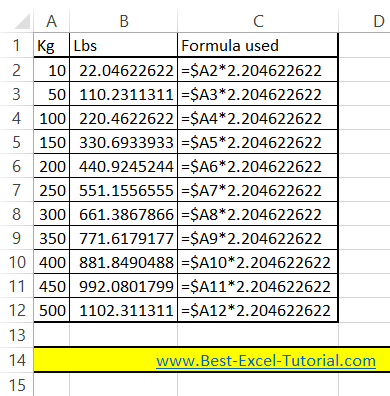Examples:

10 kg  = 10 * 2.204622622lbs = 22.04622622lbs

10 lbs = 10 * 0.45359237kg = 4.5359237kg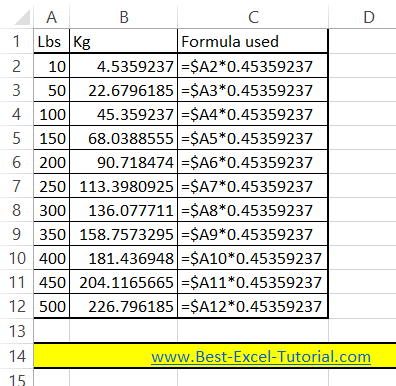Note: You may want to round the lbs value for simplification. Just use the ROUND Excel function.

10kg = ROUND(10*0.45359237,2) = 4.54lbs

250kg = ROUND(250*0.45359237,2) = 113.4lbs

500kg = ROUND(500*0.45359237,2) = 226.8lbs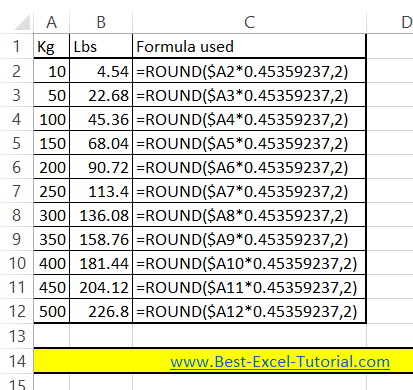## Method 2. Conversion using CONVERT Excel function

=CONVERT(cell,”unit1″,”unit2″)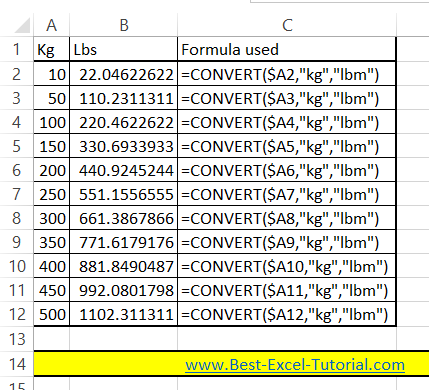Pounds are lbm in these Excel formulas.

To convert kg to lbs use:

=CONVERT(cell,”kg”,”lbm”)

To convert lbs to kg use:

=CONVERT(cell,”lbm”,”kg”)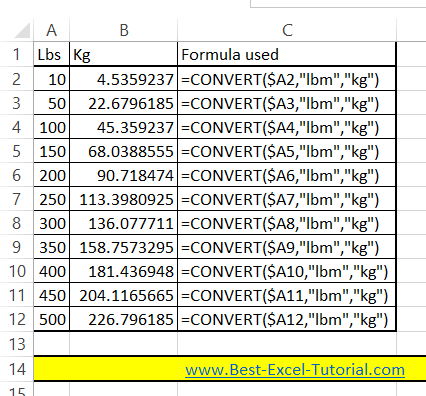## Method 3. Convert using VBA code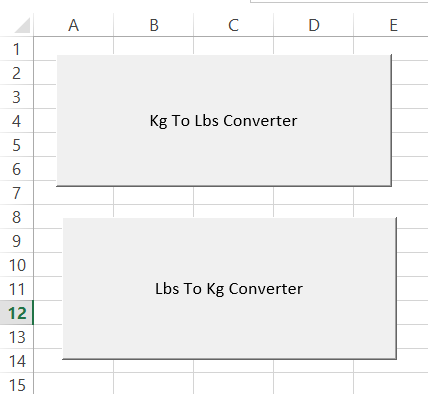This VBA code will ask you how many kg you want to convert to lbs: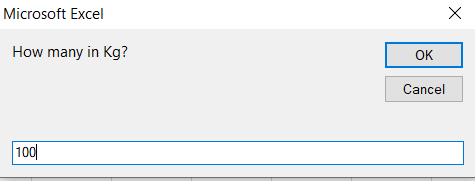```Sub KgToLbsConverter()
Dim KgToLbs As Long
KgToLbs = InputBox("How many in Kg?")
MsgBox KgToLbs * 2.204622622 & " lbs."
End Sub```

MsgBox will show you the answer.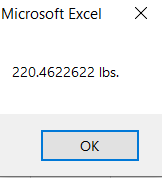This VBA code will ask you how many pounds you want to convert to kg:

```Sub LbsToKgConverter()
Dim LbsToKg As Long
LbsToKg = InputBox("How many in lbs?")
MsgBox LbsToKg * 0.45359237 & " kg."
End Sub```

MsgBox will show you the answer.

## Method 4. Convert kg to lbs and oz.

1 kg is equal to 1000 grams to it is obvious to calculate. One lb is 16 oz, making it more difficult to calculate. Luckily, there is an Excel formula which converts kg to lbs and oz.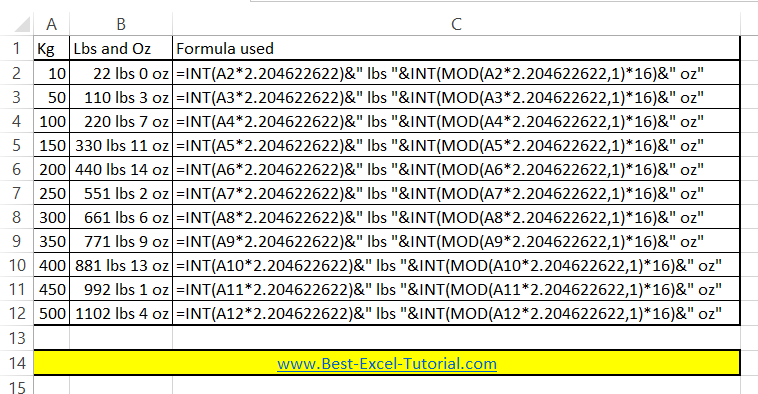=INT(A1*2.204622622)&” lbs “&INT(MOD(A1*2.204622622,1)*16)&” oz”

It will convert 250kg to 551 lb 2 oz

## Method 5. Convert kg to stones, lbs and oz.

We can go even further. We also prepared a formula which will convert kg to stones, lbs and oz. The stone is equal to 14 pounds, so it is quite easy to develop the Excel formula as follows.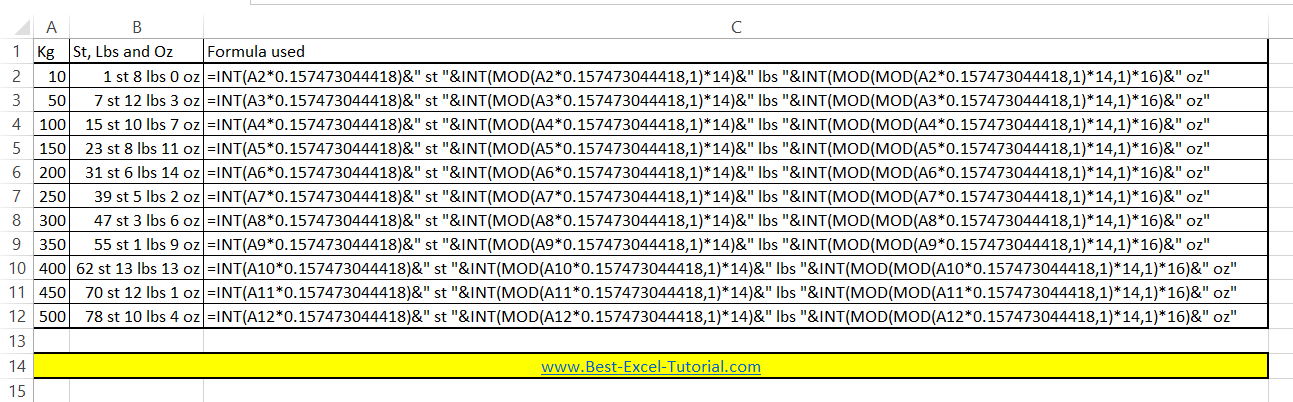=INT(A1*0.157473044418)&” st “&INT(MOD(A1*0.157473044418,1)*14)&” lbs “&INT(MOD(MOD(A1*0.157473044418,1)*14,1)*16)&” oz”

It will convert 250kg to 39 st 5 lbs 2 oz